# Area of a Triangle

In this worksheet students will learn the formula for finding the area of a triangle and use it to calculate areas.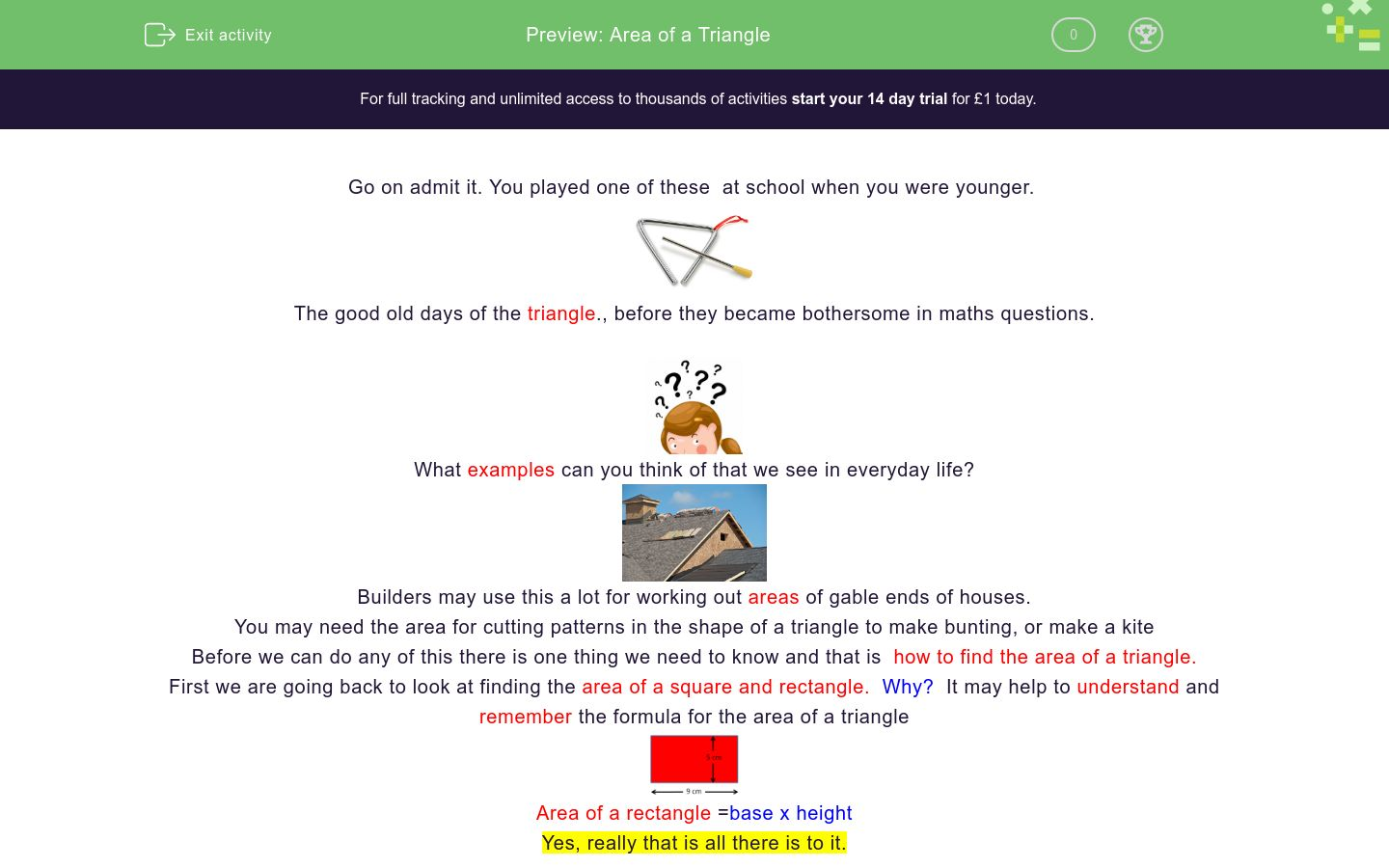Key stage:  KS 4

GCSE Subjects:   Maths

GCSE Boards:   AQA, Eduqas, Pearson Edexcel, OCR

Curriculum topic:   Geometry and Measures, Mensuration

Curriculum subtopic:   Mensuration and Calculation, Area Calculations

Difficulty level:### QUESTION 1 of 10

Go on admit it. You played one of these  at school when you were younger.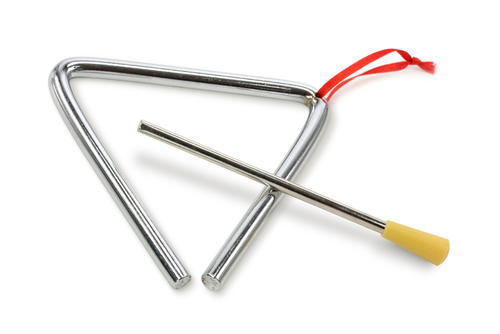The good old days of the triangle., before they became bothersome in maths questions.What examples can you think of that we see in everyday life?Builders may use this a lot for working out areas of gable ends of houses.

You may need the area for cutting patterns in the shape of a triangle to make bunting, or make a kite

Before we can do any of this there is one thing we need to know and that is  how to find the area of a triangle.

First we are going back to look at finding the area of a square and rectangle.  Why?  It may help to understand and remember the formula for the area of a triangleArea of a rectangle =base x height

Yes, really that is all there is to it.Look what happens when a diagonal line is drawn from one corner of the rectangle to the other.  By cutting it in half I have made triangles. Cutting it in half is the same as dividing by 2.

Area = base x height ÷ 2

or another way of writing this is

Area = 1/2 base x heightNow you could go barking up the wrong tree so be careful.

When you are given measurements for a triangle you are sometimes given the base, vertical height and a slanted height.

You always want the vertical height.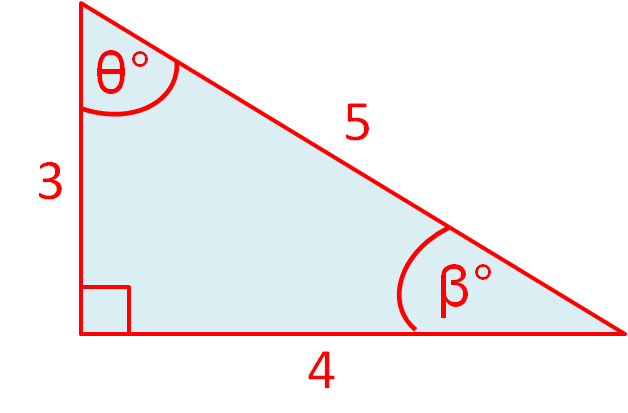The area of this triangle  is the base of 4 x vertical height of 3 = 12

12 ÷ 2 = 6 units²

Once you have learned the formula you are flying.Find the area of this triangle.

108 cm²

11 cm²

54 ²

11.5 cm²

Match these areas

## Column B

Triangle base 4 cm height 3cm
21 cm²
Triangle base 5 cm height 10 cm
32 cm²
Triangle base 8 cm height 8 cm
12 cm²
Triangle base 12 cm height 2 cm
66 cm²
Triangle base 11 cm height 12 cm
6 cm²
Triangle base 6 cm height 7 cm
25 cm²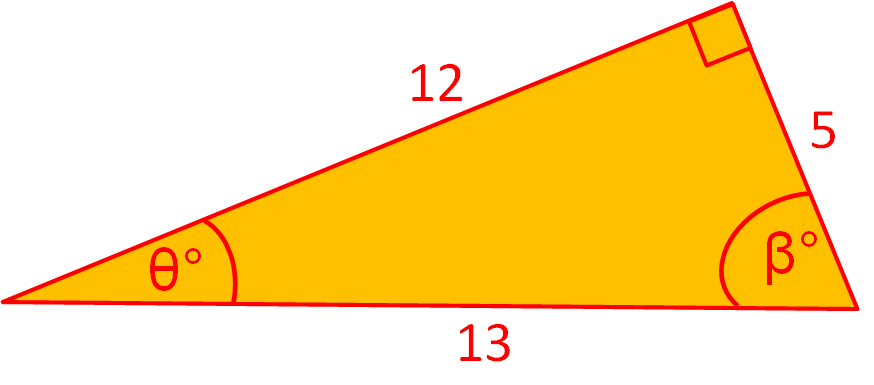Find the area of this triangle.The slices of toast have a side of 14 cm.

You are going to cut the toast into triangles.  What is the area of one triangle of toast?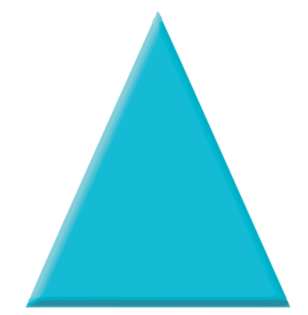This triangle  has a base of 10 cm and a height of 42 mm.  Calculate its area.

21 cm²

420 mm²

2100 mm²

210 cm²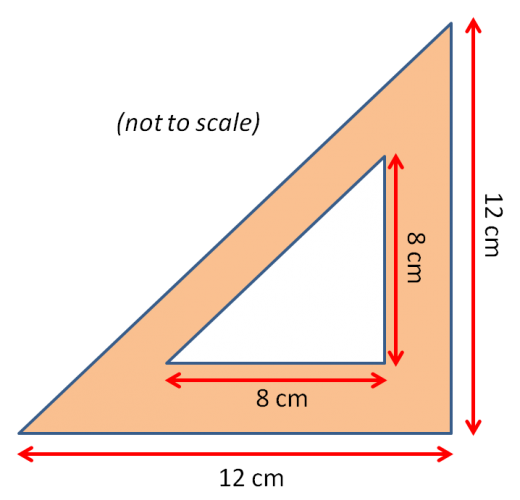What is the difference in area between the orange triangle and the white triangle?

80 cm²

32 cm²

40 cm²

35 cm²This warning triangle has as base of  30 cm and a height of 40 cm.

10 of these triangles are needed for a factory floor.  What is the total area of metal used in making these warning triangles.The base of this triangle measures x and the height is x + 4.  What is its area?

There are two ways to write this.

2x² + 2 x÷ 2

(x) x (x + 4) ÷ 2

x² + 4 x÷ 2

2x² + 4 x ÷ 2The base of this triangle measures x  + 3 and the height is x + 4.  What is its area?

There are two possible ways to write this answer.

(x + 3) x (x + 4) ÷ 2

12x² ÷ 2

x² + 7x + 12 ÷ 2

2x + 7 ÷2;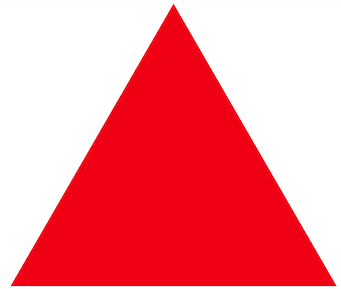The area of this triangle is 40 cm²  If the height is 8 cm, calculate the base.

• Question 1Find the area of this triangle.

54 ²
EDDIE SAYS
Did you apply the formula? Base x height ÷ 2 12 x 9 = 108 cm² 108 cm² = 54 cm² It is easy to forget the second step so keep practising
• Question 2

Match these areas

## Column B

Triangle base 4 cm height 3cm
6 cm²
Triangle base 5 cm height 10 cm
25 cm²
Triangle base 8 cm height 8 cm
32 cm²
Triangle base 12 cm height 2 cm
12 cm²
Triangle base 11 cm height 12 cm
66 cm²
Triangle base 6 cm height 7 cm
21 cm²
EDDIE SAYS
Applying the formula is now getting easy. Keep remembering the divide by 2. base times height divide by two should be your mantra. 4 x 3 = 12 ÷ 2 = 6 5 x 10 = 50 ÷ 2 = 25 8 x 8 = 64 ÷ 2 = 32 12 x 2 = 24 ÷ 2 = 12 11 x 12 = 132 ÷ 2 = 66 6 x 7 = 142 ÷ 2 = 21 Not forgetting units squared.
• Question 3Find the area of this triangle.

30
EDDIE SAYS
Did you remember to select the vertical height? The only thing you need from this diagram is the base and vertical height. Now this is not always easy to spot, if the triangle has been flipped round. The base here is 5cm the vertical height is 12 cm 5 x 12 = 60 60 ÷: 2 = 30 cm²
• Question 4The slices of toast have a side of 14 cm.

You are going to cut the toast into triangles.  What is the area of one triangle of toast?

98
EDDIE SAYS
We know the area of a square is base x height. We know the sides are the same 14 x 14 = 196 Cut the toast in half to make triangles 196 ÷ 2 = 98 cm&2; Don't burn the toast worrying about working out the area.
• Question 5This triangle  has a base of 10 cm and a height of 42 mm.  Calculate its area.

21 cm²
2100 mm²
EDDIE SAYS
Did you spot the two different units? You can convert them either way 10 x 4.2 = 42 m² ÷ 2 = 21 cm² 100 x 42 = 4200 mm² ÷ 2 = 2100 mm²
• Question 6What is the difference in area between the orange triangle and the white triangle?

40 cm²
EDDIE SAYS
This is just a 3 step question. You have all the knowledge that you need. Area of orange triangle = 12 x 12 = 144 cm² ÷ 2 = 72 cm² Area of white triangle = 8 x 8 = 64 cm² ÷ 2 = 32 cm² 72 - 32 = 40 cm² Just think they are triangles, so three steps
• Question 7This warning triangle has as base of  30 cm and a height of 40 cm.

10 of these triangles are needed for a factory floor.  What is the total area of metal used in making these warning triangles.

6000
EDDIE SAYS
This is not as hazardous as you might think. Find the area of one triangle and multiply by 10. 30 x 40 = 1200 1200 ÷ 2 = 600 600 x 10 = 6000 cm²
• Question 8The base of this triangle measures x and the height is x + 4.  What is its area?

There are two ways to write this.

(x) x (x + 4) ÷ 2
x² + 4 x÷ 2
EDDIE SAYS
A curved ball, don't your just love them In some ways these are easier because you don't know the value of x. All you can do is write what you know (x) multiplied by (x + 4) or x multiplied by x = x² x multiplied by 4 = 4x so x² + 4x ÷ 2
• Question 9The base of this triangle measures x  + 3 and the height is x + 4.  What is its area?

There are two possible ways to write this answer.

(x + 3) x (x + 4) ÷ 2
x² + 7x + 12 ÷ 2
EDDIE SAYS
And another just for fun. In some ways these are easier because you don't know the value of x. All you can do is write what you know so (x + 3) x (x + 4) ÷ 2 You could multiply out the brackets and simplify x² + 7x + 12 ÷ 2
• Question 10The area of this triangle is 40 cm²  If the height is 8 cm, calculate the base.

10 cm
EDDIE SAYS
Okay just when you thought you had everything under control. You have all the information you need. Area = base x height ÷2; Substitute what you know into the formula 40 cm² = ? x h ÷2; Work backwards 40 ÷ H 40 ÷: 8 = 5 5 x 2 = 10cm Test it out! 8 x 10 = 80 ÷ 2 = 40 cm²
---- OR ----

Sign up for a £1 trial so you can track and measure your child's progress on this activity.

### What is EdPlace?

We're your National Curriculum aligned online education content provider helping each child succeed in English, maths and science from year 1 to GCSE. With an EdPlace account you’ll be able to track and measure progress, helping each child achieve their best. We build confidence and attainment by personalising each child’s learning at a level that suits them.

Get started